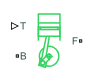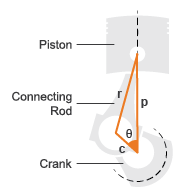# Piston

Piston mechanism of reciprocating combustion engine

•Libraries:
Simscape / Driveline / Engines & Motors

## Description

The Piston block represents the piston mechanism of a reciprocating combustion engine. The Piston block accounts for the instantaneous torque transmitted to the engine crankshaft, which enables you to simulate vibrations in the drivetrain due to piston revolution. To model a multi-piston engine, use the Piston Engine block.

Port B represents the translating piston and port F represents the rotating crankshaft. The piston force follows from the cylinder pressure and cross-sectional area. The block obtains the combustion pressure from a lookup table parameterized in terms of the crank angle and, optionally, the crank angular velocity and engine throttle level.

The crank torque follows from the piston force and crank angle, the crank and connecting rod lengths. In terms of these inputs, the ratio of the piston force and crank torque is

`$\frac{{T}_{\text{F}}}{{F}_{\text{B}}}=-\text{c}\left(sin\left(\theta \right)+\frac{sin\left(2\theta \right)}{2\sqrt{{\left(\frac{\text{r}}{\text{c}}\right)}^{2}-{\mathrm{sin}}^{2}\left(\theta \right)}}\right),$`

where:

• FB is the instantaneous piston force associated with the base port.

• TF is the instantaneous crank torque associated with the follower port.

• c is the crank length.

• θ is the instantaneous crank angle.

• r is the connecting rod length.

Piston DimensionsPort T lets you specify the engine throttle level as a fraction between 0 and 1. This fraction corresponds to the percentage of full power generated. The block uses the data at point T whenever the pressure lookup table in the block property inspector is parameterized only in terms of the crank angle.

## Ports

### Input

expand all

Physical signal input port that specifies engine throttle setting.

### Output

expand all

Physical signal output port that returns the fuel consumption rate.

#### Dependencies

To enable this port, set Fuel consumption model to one of the following.

• `Constant per revolution`

• ```Fuel consumption by speed and torque```

• ```Brake specific fuel consumption by speed and torque```

• ```Brake specific fuel consumption by speed and brake mean effective pressure```

### Conserving

expand all

Translational mechanical conserving port that connects to the engine block.

Rotational mechanical conserving port that connects to the engine crankshaft.

## Parameters

expand all

### Main

Inside diameter of the piston cylinder wall. The Piston block uses this measurement to compute the torque table. You must specify a value greater than zero.

Distance from the fully retracted position to the fully extended position of the piston. The Piston block uses this measurement to convert pressure on the piston into torque values. You must specify a value greater than zero.

Distance from the center of the piston pin hole to the center of the crankshaft hole in the piston rod. The block uses this measurement to convert pressure on the piston into torque. You must specify a value greater than zero.

Number of piston phases required to intake, compress, combust, and exhaust the combustion gases. Typically, engines are two-stroke or four-stroke. You must use a multiple of two.

Parameter group that you want the block to use to parameterize the pressure data.

Angle of the piston crank starting at or above the minimum angle and ending at or below the maximum angle. You can calculate the minimum or maximum angle by multiplying the number of strokes by -90 degrees or 90 degrees, respectively. You must specify at least two values.

Each element in Crank angle vector corresponds to an element in Pressure vector (gauge) or the M-row of Pressure matrix (gauge) or each Pressure 3D matrix (gauge) matrix.

Different throttle positions that correspond to the pressures in the Pressure matrix (gauge) or Pressure 3D matrix (gauge) parameters. The throttle position must remain in the range [0,1] with 0 representing no throttle and 1 representing full throttle.

Each element in Throttle vector corresponds to the N-column of Pressure matrix (gauge) or each Pressure 3D matrix (gauge) matrix.

#### Dependencies

To enable this parameter, set Pressure parameterization to ```By crank angle and throttle``` or ```By crank angle, throttle, and crank velocity```.

Different crank velocities that correspond to pressures in Pressure 3D matrix (gauge).

Each element in Crank velocity vector corresponds to one of the O-matrices of Pressure 3D matrix (gauge).

#### Dependencies

To enable this parameter, set Pressure parameterization to ```By crank angle, throttle, and crank velocity```.

Vector of pressure values that correspond to different crank angle positions.

#### Dependencies

To enable this parameter, set Pressure parameterization to ```By crank angle```.

Matrix of pressure values that correspond to different crank angle and throttle combinations. The default value is ```[0, 0, 0, 0; 0, .9, 2.4, 3; 0, 6, 16, 20; 0, 15, 40, 50; 0, 6, 16, 20; 0, 3, 8, 10; 0, 2.4, 6.4, 8; 0, 0, 0, 0]``` `bar`.

The elements of Crank angle vector correspond to the M-rows in Pressure matrix (gauge). The elements of Throttle vector correspond to the N-columns in Pressure matrix (gauge).

#### Dependencies

To enable this parameter, set Pressure parameterization to ```By crank angle and throttle```.

Concatenated matrix of pressure values that correspond to the various crank angle, throttle, and crank velocity combinations. The default value is ```cat(3, [0, 0, 0, 0; 0, .9, 2.4, 3; 0, 6, 16, 20; 0, 15, 40, 50; 0, 6, 16, 20; 0, 3, 8, 10; 0, 2.4, 6.4, 8; 0, 0, 0, 0], [0, 0, 0, 0; 0, .9, 2.4, 3; 0, 6, 16, 20; 0, 15, 40, 50; 0, 6, 16, 20; 0, 3, 8, 10; 0, 2.4, 6.4, 8; 0, 0, 0, 0], [0, 0, 0, 0; 0, .9, 2.4, 3; 0, 6, 16, 20; 0, 15, 40, 50; 0, 6, 16, 20; 0, 3, 8, 10; 0, 2.4, 6.4, 8; 0, 0, 0, 0])``` `bar`.

The elements of Crank angle vector correspond to the M-rows. The elements of Throttle vector correspond to the N-columns. The elements of Crank velocity vector correspond to the matrices you concatenate along dimension O.

By default, M = 8, N = 4, and O = 3.

#### Dependencies

To enable this parameter, set Pressure parameterization to ```By crank angle, throttle, and crank velocity```.

Aggregate viscous friction coefficient of the main and rod bearings. The block uses this parameter to compute the viscous power losses between the base and follower shafts.

Point in the engine cycle when the piston reaches top dead center. The engine cycle spans in angle from -S*180 to +S*180 degrees, where S is the value of the Number of strokes per cycle parameter.

Crank angle at time zero relative to a top dead center position.

### Fuel Consumption

Model for calculating fuel consumption. Model parameterizations are compatible with standard industrial data.

Selecting any option other than ```No fuel consumption``` enables the FC port and related parameters. When you set Fuel consumption model to `No fuel consumption`, the block does not calculate fuel consumption. Selecting this option increases simulation speed.

Volume of fuel consumed in one crankshaft revolution.

#### Dependencies

To enable this parameter, set Fuel consumption model to ```Constant per revolution```.

Vector of engine speeds used in lookup table parameterizations. The vector length must match the Torque vector.

#### Dependencies

To enable this parameter, set Fuel consumption model to one of these settings:

• ```Fuel consumption by speed and torque```

• ```Brake specific fuel consumption by speed and torque```

• ```Brake specific fuel consumption by speed and brake mean effective pressure```

Vector of engine torques used in the lookup table parameterizations. The vector length must match the Speed vector parameter.

#### Dependencies

To enable this parameter, set Fuel consumption model to ```Fuel consumption by speed and torque``` or ```Brake specific fuel consumption by speed and torque```.

Matrix of fuel consumption rates corresponding to engine speed and torque vectors. The number of rows, P, must equal the number of elements in the Speed vector parameter. The number of columns, Q, must equal the number of elements in the Torque vector parameter. The default value is ```[.5, .9, 1.4, 1.6, 1.9, 2.7, 3.4, 4.4; 1, 1.7, 2.7, 3.1, 3.6, 5, 6, 7.4; 1.4, 2.7, 4, 4.8, 5.6, 7.5, 8.5, 10.5; 2, 3.6, 5.8, 6.7, 8, 10.4, 11.7, 13.3; 2.5, 4.8, 7.9, 9.4, 10.8, 14, 16.2, 18.6; 3.1, 6, 10.3, 11.9, 13.8, 18.4, 22, 26.5]``` `g/s`.

#### Dependencies

To enable this parameter, set Fuel consumption model to ```Fuel consumption by speed and torque``` or ```Brake specific fuel consumption by speed and torque```.

Vector of brake mean effective pressure (BMEP) values. The BMEP satisfies the expression:

`$BMEP=T\cdot \left(\frac{2\pi \cdot {n}_{c}}{{V}_{d}}\right),$`

where:

• T is the output torque.

• nc is the number of cycles per revolution.

• Vd is the cylinder displaced volume.

#### Dependencies

To enable this parameter, set Fuel consumption model to ```Fuel consumption by speed and torque``` or ```Brake specific fuel consumption by speed and brake mean effective pressure```.

Matrix of brake specific fuel consumption (BSFC) rates. BSFC is the ratio of the fuel consumption rate to the output power. The number of rows, P, must equal the number of elements in the Speed vector parameter. The number of columns, Q, must equal the number of elements in the Torque vector parameter. The default value is ```[410, 380, 300, 280, 270, 290, 320, 380; 410, 370, 290, 270, 260, 270, 285, 320; 415, 380, 290, 275, 265, 270, 270, 300; 420, 390, 310, 290, 285, 280, 280, 285; 430, 410, 340, 320, 310, 300, 310, 320; 450, 430, 370, 340, 330, 330, 350, 380]``` `g/hr/kW`.

When you set Fuel consumption model to ```Brake specific fuel consumption by speed and torque```, enter a matrix with BSFC rates corresponding to engine speed and torque vectors.

When you set Fuel consumption model to ```Brake specific fuel consumption by speed and brake mean effective pressure```, enter a matrix with BSFC rates corresponding to engine speed and brake mean effective pressure (BMEP) vectors.the number of rows must equal the number of elements in the Speed vector parameter. The number of columns must equal the number of elements in the Brake mean effective pressure vector parameter.

#### Dependencies

To enable this parameter, set Fuel consumption model to ```Brake specific fuel consumption by speed and torque``` or ```Brake specific fuel consumption by speed and brake mean effective pressure```.

Interpolation method to calculate fuel consumption at intermediate speed and torque values. Outside the data range, fuel consumption remains constant at the last value given in the lookup table for both methods.

#### Dependencies

To enable this parameter, set Fuel consumption model to one of these settings:

• ```Fuel consumption by speed and torque```

• ```Brake specific fuel consumption by speed and torque```

• ```Brake specific fuel consumption by speed and brake mean effective pressure```

## Version History

Introduced in R2016a